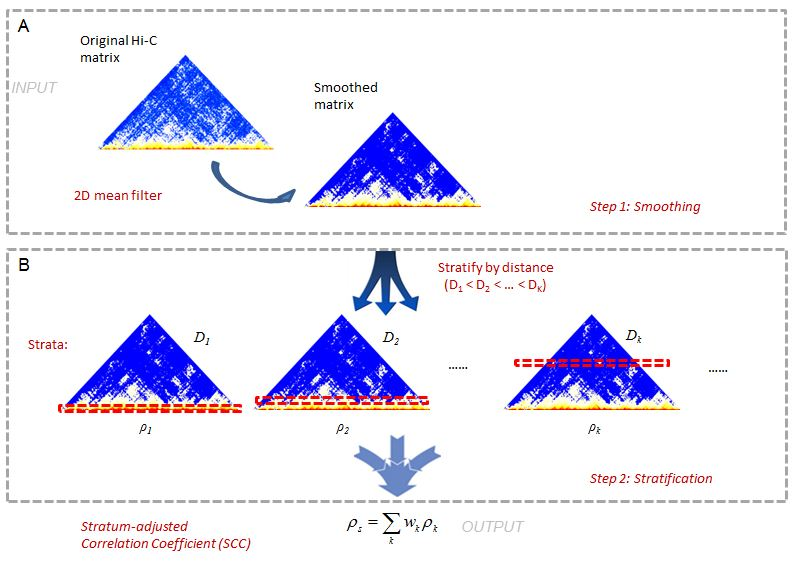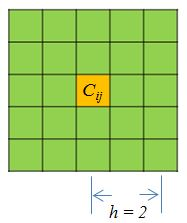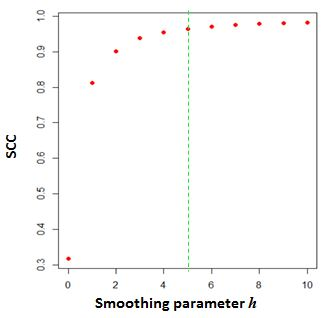# Evaluate reproducibility of Hi-C data with hicrep" In hicrep: Measuring the reproducibility of Hi-C data

## Introduction

Hi-C data analysis and interpretation are still in their early stages. In particular, there has been a lack of sound statistical metric to evaluate the quality of Hi-C data. When biological replicates are not available, investigators often rely on eithervisual inspection of Hi-C interaction heatmap or examining the ratio of long-range interaction read pairs over the total sequenced reads, neither of which are supported by robust statistics. When two or more biological replicates are available, it is a common practice to compute either Pearson or Spearman correlation coefficients between the two Hi-C data matrices and use them as a metric for quality control. However, these kind of over-simplified approaches are problematic and may lead to wrong conclusions, because they do not take into consideration of the unique characteristics of Hi-C data, such as distance-dependence and domain structures. As a result, two un-related biological samples can have a strong Pearson correlation coefficient, while two visually similar replicates can have poor Spearman correlation coefficient. It is also not uncommon to observe higher Pearson and Spearman correlations between unrelated samples than those between real biological replicates.

we develop a novel framework, hicrep, for assessing the reproducibility of Hi-C data. It first minimizes the effect of noise and biases by smoothing Hi-C matrix, and then addresses the distance-dependence effect by stratifying Hi-C data according to their genomic distance. We further adopt a stratum-adjusted correlation coefficient (SCC) as the measurement of Hi-C data reproducibility. The value of SCC ranges from -1 to 1, and it can be used to compare the degrees of differences in reproducibility. Our framework can also infer confidence intervals for SCC, and further estimate the statistical significance of the difference in reproducibility measurement for different data sets.

In this Vignette, we explain the method rationale, and provide guidance to use the functions of hicrep to assess the reproducibility for Hi-C intrachromosome replicates.

## Citation

Cite the following paper:

HiCRep: assessing the reproducibility of Hi-C data using a stratum-adjusted correlation coefficient. Tao Yang, Feipeng Zhang, Galip Gurkan Yardimci, Ross C Hardison, William Stafford Noble, Feng Yue, Qunhua Li. bioRxiv 101386; doi: https://doi.org/10.1101/101386

## Installation

Download the source package hicrep_0.99.5.tar.gz from Github. Or install it from Bioconductor.

## Rationale of method

This is a 2-step method (Figure1). In Hi-C data it is often difficult to achieve sufficient coverage. When samples are not sufficiently sequenced, the local variation introduced by under-sampling can make it difficult to capture large domain structures. To reduce local variation, we first smooth the contact map before assessing reproducibility. Although a smoothing filter will reduce the individual spatial resolution, it can improve the contiguity of the regions with elevated interaction, consequently enhancing the domain structures. We use a 2D moving window average filter to smooth the Hi-C contact map. This choice is made for the simplicity and fast computation of mean filter, and the rectangular shape of Hi-C compartments.

In the second step, we stratify the Hi-C reads by the distance of contacting loci, calculate the Pearson correlations within each stratum, and then summarize the stratum-specific correlation coefficients into an aggregated statistic. We name it as Stratum-adjusted Correlation Coefficient (SCC). For the methodology details, please refer to our manuscript.knitr::opts_knit$set(progress = TRUE, verbose = TRUE) library(hicrep) data("HiCR1") data("HiCR2")  ## The format of input and Pre-processing The input are simply two Hi-C matrices to be compared. The Hi-C matrices should have the dimension$N\times(3+N)$. The three additional initial columns are the chromosome name and mid-point coordinates of the two contact bins. Here, we should a example data: dim(HiCR1) HiCR1[1:10,1:10]  A single function prep will tranform the HiC matrix, smooth the data with given neighborhood size parameter$h$, and filter the bins that have zero counts in both replicates. The arguments includes the two matrices, the resolution of matrices, smoothing parameter, and the maximum distance considered. The resolution is simply the bin size. Smoothing parameter decides the neighborhood size of smoothing. Below (Figure 2) is a demonstration of smoothing neighborhood for a point$C_{ij}$:The maximum distance is that contacts beyond this distance will be under investigation. Here we are showing an example that has resolution 40kb, smoothing parameter$h = 1$, and contacts with distance over 5M will not be considered: Pre_HiC <- prep(HiCR1, HiCR2, 1000000, 1, 5000000) head(Pre_HiC)  The first two columns of the output are the mid-point coordinates of two contact bins, and the last two are the smoothed (or not if$h = 0$) values of the two replicates. ## Select the optimal smoothing parameter A natrual question is how to select the smoothing parameter$h$.To select$h$objectively, we develop a heuristic procedure to search for the optimal smoothing parameter. Our procedure is designed based on the observation that the correlation between contact maps of replicate samples first increases with the level of smoothness and plateaus when sufficient smoothness is reached. To proceed, we use a pair of reasonably deeply sequenced interaction map as the training data. We randomly partition the data into ten fractions 10% of the data, then compute SCC for the sampled dataeach fraction at a series of smoothing parameters in the ascending order, then choose the smallest h at which the increment of the average reproducibility score is less than 0.01. This procedure is repeated ten times, and the mode among the ten h’s is picked. h_hat <- htrain(HiCR1, HiCR2, 1000000, 5000000, 0:2) h_hatThe above graph shows the change of SCC as the$h$increases from 0 to 10. The parameter$h=5\$ is selected as the optimal smoothing neighborhood size.

The smoothing parameter selection could be confounded by the sequencing depth. Insufficient sequencing depth data might lead to inflated smoothing neighborhood size. We suggest to use sufficient sequenced Hi-C data to train the smoothing parameter given the resolution (i.e., > 300 million total nubmer of reads for whole chromosome). To compare SCC between pairs of replicates that has the same resolution, one shall use the same smoothing parameter.

## Equalize the total number of reads

In previous section, we mention that sequencing depth could be a confounding effect. If the total numbers of reads are very different between the two replicates, it's suggested that one should down-sample the higher sequencing depth to make it equal the lower one. The best way to do it is to use the bam files to do the sub-sampling. In case you only have the matrix file available, we made a function to do down-sampling from matrix files.

#check total number of reads before adjustment
sum(HiCR1[,-c(1:3)])

DS_HiCR1 <- depth.adj(HiCR1, 200000, 1000000, out = 0)

sum(DS_HiCR1[,-c(1:3)])


When out = 0, the function will return matrix in the original format. When it equals 1, the vectorized format will be outputed.

## Calculate Stratum-adjusted Correlation Coefficient (SCC)

After the raw matrices are pre-processed, we are ready to calculate the SCC and its variance. The input file is just the output dataset (i.e., vectorized, smoothed, zero-in-both filtered) from the function prep. We also need to specify the resolution of the matrix, and the maximum contacting distance considered.

SCC.out = get.scc(Pre_HiC, 1000000, 5000000)

#SCC score
SCC.out[]

#Standard deviation of SCC
SCC.out[]
sessionInfo()


The output is a list of results including stratum specific Pearson correlations, weight coefficient, SCC and the asymptotic standard deviation of SCC. The last two numbers are the ones we needed in most of the situations.

## Computation efficiency

The computation speed is determined by the size of the chromosome. For the chromosome 22 data, htrain needs approximately 15 minutes, and the get.scc takes about 1~2 minutes. For chromosome 1, get.scc takes about 90 minutes. the The estimation is based on Intel i7-3250M core.

## Try the hicrep package in your browser

Any scripts or data that you put into this service are public.

hicrep documentation built on April 28, 2020, 7:51 p.m.